# HSSlive: Plus One & Plus Two Notes & Solutions for Kerala State Board

## AP Board Class 7 Maths Chapter 8 Congruency of Triangles Ex 4 Textbook Solutions PDF: Download Andhra Pradesh Board STD 7th Maths Chapter 8 Congruency of Triangles Ex 4 Book AnswersAP Board Class 7 Maths Chapter 8 Congruency of Triangles Ex 4 Textbook Solutions PDF: Download Andhra Pradesh Board STD 7th Maths Chapter 8 Congruency of Triangles Ex 4 Book Answers

## Andhra Pradesh State Board Class 7th Maths Chapter 8 Congruency of Triangles Ex 4 Books Solutions

 Board AP Board Materials Textbook Solutions/Guide Format DOC/PDF Class 7th Subject Maths Chapters Maths Chapter 8 Congruency of Triangles Ex 4 Provider Hsslive

2. Click on the Andhra Pradesh Board Class 7th Maths Chapter 8 Congruency of Triangles Ex 4 Answers.
3. Look for your Andhra Pradesh Board STD 7th Maths Chapter 8 Congruency of Triangles Ex 4 Textbooks PDF.
4. Now download or read the Andhra Pradesh Board Class 7th Maths Chapter 8 Congruency of Triangles Ex 4 Textbook Solutions for PDF Free.

## AP Board Class 7th Maths Chapter 8 Congruency of Triangles Ex 4 Textbooks Solutions with Answer PDF Download

Find below the list of all AP Board Class 7th Maths Chapter 8 Congruency of Triangles Ex 4 Textbook Solutions for PDF’s for you to download and prepare for the upcoming exams:

Question 1.
Which congruence criterion do you use in the following?
(i) Given :AC = DF
AB = DE
BC = EF
So, ΔABC ≅ ΔDEF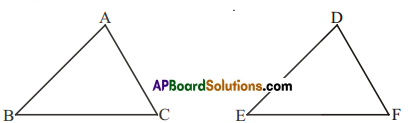(ii) Given: ZX = RP
RQ = ZY
∠PRQ ≅ ∠XZY
So, ΔPQR ≅ ΔXYZ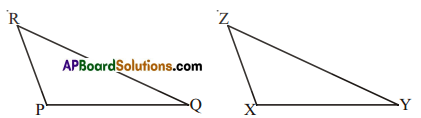(iii) Given: ∠MLN ≅∠FGH
∠NML ≅ ∠GFH
ML = FG
So, ΔLMN ≅ ΔGFH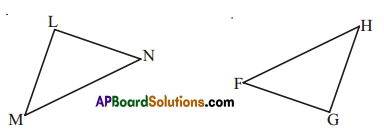(iv) Given: EB = DB . D
AE = BC
∠A = ∠C = 90°
So, ΔABE ≅ ΔCDB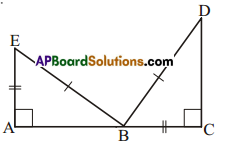Solution:
(i) S.S.S congruence
(ii) S.A.S congruence
(iii) A.S.A congruence
(iv) By R.H.S congruence

Question 2.
You want to show that ΔART ≅ ΔPEN,
(i) If you have to use SSS criterion, then you need to show
(a) AR= (b) RT = (c) AT=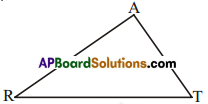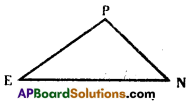Solution:
(i) (a) AR = PE
(b) RT = EN
(c) AT = PN

(ii) If it is given that ∠T = ∠N and you are to use SAS criterion, you need to have
(a) RT = ? and (ii) PN = ?
Solution:
(a) RT = EN and
(ii) PN = AT

(iii) If it is given that AT = PN and you arelo use ASA criterion, you need to have
(a)? (b)?
Solution:
a) ∠A = ∠P
b) ∠T = ∠N

Question 3.
You have to show that ΔAMP ≅ ΔAMQ. In the following proof. supply the missing reasons.

 Steps Reasons (i) PM = QM (i) ……………. (ii) ∠PMA ≅ ∠QMA (ii) ……………. (iii) AM = AM (iii) ……………. (iv) ∆AMP ≅ ∆AMQ (iv) …………….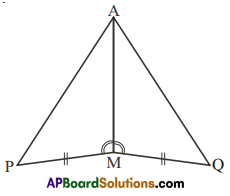Solution:

 Steps Reasons (i) PM=QM (i) Given side (ii) ∠PMA ≅ ∠QMA (ii) Given angle (iii) AM=AM (iii) Common side (iv) ∆AMP ≅ ∆AMQ (iv) A.A.S criterion

Question 4.
In ΔABC’, ∠A = 30°, ∠B = 40° and ∠C = 110°
In ΔPQR, ∠P = 30°, ∠Q = 40°and ∠R = 110°
A student says that ΔABC ≅ ΔPQR by AAA congruence criterion. Is hejustified? Why or why not?
Solution:
The student Is not correct.
AAA is not a congruency criteria.
Triangles with same corresponding angles can have different sizes.

Question 5.
In the figure, the two triangles are congruent. The corresponding parts are marked. We can write ΔRAT? ≅ ?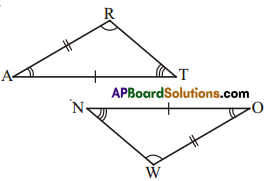Solution:
ΔRAT ≅ ΔWON

Question 6.
Complete the congruence statement.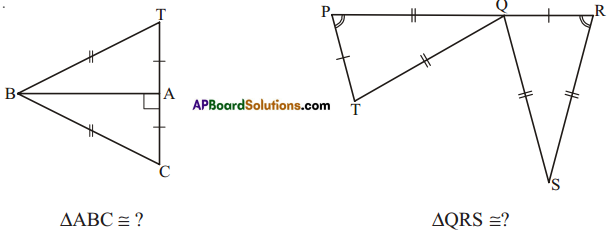Solution:
ΔABC ≅ ?
ΔABC ≅ ΔABT

ΔQRS ≅ ?
ΔQRS ≅ ΔTPQ

Question 7.
In a squared sheet, draw two triangles of equal areas such that
(i) the triangles are congruent.
(ii) the triangles are not congruent.
What can you say about their perimeters?
Solution: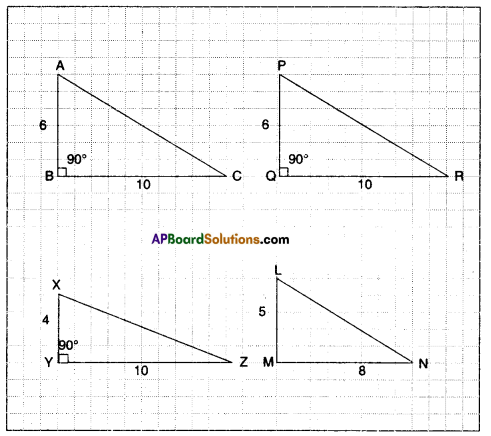i) ΔABC ≅ ΔPQR
Since AB = PQ
∠B = ∠Q
BC = QR (Perimeter is same)

ii) Area of ΔXYZ = 12 x 10 x 4 : 20 sq.units.
Areas of ΔLMN= 12 x 8 x 5=2Osq. units
But ΔXYZ and ΔLMN are not congruent.
(Perimeter is different)

Question 8.
If ΔABC and ΔPQR are to be congruent, name one additional pair of corresponding parts. What criterion did you use?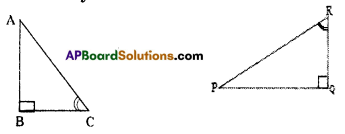Solution:
We need to know that BC = QR. Here we use A.S.A criterion.

Question 9.
Explain, why
ΔABC ≅ ΔFED.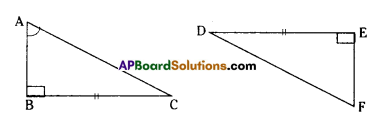Solution:
∠B = ∠E
BC = ED
∠C = ∠D by angle sum property.

## Andhra Pradesh Board Class 7th Maths Chapter 8 Congruency of Triangles Ex 4 Textbooks for Exam Preparations

Andhra Pradesh Board Class 7th Maths Chapter 8 Congruency of Triangles Ex 4 Textbook Solutions can be of great help in your Andhra Pradesh Board Class 7th Maths Chapter 8 Congruency of Triangles Ex 4 exam preparation. The AP Board STD 7th Maths Chapter 8 Congruency of Triangles Ex 4 Textbooks study material, used with the English medium textbooks, can help you complete the entire Class 7th Maths Chapter 8 Congruency of Triangles Ex 4 Books State Board syllabus with maximum efficiency.

## FAQs Regarding Andhra Pradesh Board Class 7th Maths Chapter 8 Congruency of Triangles Ex 4 Textbook Solutions

#### Can we get a Andhra Pradesh State Board Book PDF for all Classes?

Yes you can get Andhra Pradesh Board Text Book PDF for all classes using the links provided in the above article.

## Important Terms

Andhra Pradesh Board Class 7th Maths Chapter 8 Congruency of Triangles Ex 4, AP Board Class 7th Maths Chapter 8 Congruency of Triangles Ex 4 Textbooks, Andhra Pradesh State Board Class 7th Maths Chapter 8 Congruency of Triangles Ex 4, Andhra Pradesh State Board Class 7th Maths Chapter 8 Congruency of Triangles Ex 4 Textbook solutions, AP Board Class 7th Maths Chapter 8 Congruency of Triangles Ex 4 Textbooks Solutions, Andhra Pradesh Board STD 7th Maths Chapter 8 Congruency of Triangles Ex 4, AP Board STD 7th Maths Chapter 8 Congruency of Triangles Ex 4 Textbooks, Andhra Pradesh State Board STD 7th Maths Chapter 8 Congruency of Triangles Ex 4, Andhra Pradesh State Board STD 7th Maths Chapter 8 Congruency of Triangles Ex 4 Textbook solutions, AP Board STD 7th Maths Chapter 8 Congruency of Triangles Ex 4 Textbooks Solutions,
Share: